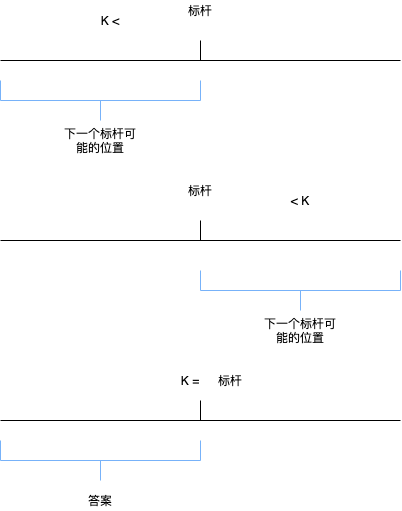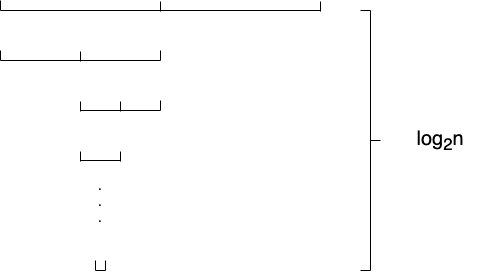# 快速筛出topK的快速选择算法和BFPRT优化

2019/04/10 10:10

<br>

<br>

<br>

<br>

## 算法原理

<br>def quick_select_without_optimizer(arr, k):
n = len(arr)
# 如果k大于n，没啥好说的，直接返回
if k >= n:
return arr

# 缓存
buffer = []
while arr:
# 选择最后一个元素作为标杆
mark = arr.pop()
less, greater = [], []
# 遍历数组，将元素分为less和greater
for x in arr:
if x <= mark:
less.append(x)
else:
greater.append(x)
# 判断三种情况，如果相等直接返回
if len(less) == k:
return less
# 如果小于，将less存入buffer，因为它一定是答案的一部分，可以简化计算
elif len(less) < k:
buffer += less
# k要减去less的长度
k -= len(less)
arr = [mark] + greater
else:
# 如果大于，直接舍弃右边
arr = less



<br>

## 复杂度分析

<br>$1, 2, 4, \cdots, n$

$\displaystyle S=\frac{a_1(1-q^n)}{1-q}=\frac{1(1-2n)}{1-2}\approx 2n$

<br>

<br>

<br>

## BFPRT算法

<br>

1. 判断数组元素是否大于5，如果小于5，对它进行排序，并返回数组的中位数
2. 如果元素大于5个，对数组进行分组，每5个元素分成一组，允许最后一个分组元素不足5个。
3. 对于每个分组，对它进行插入排序
4. 选择出每个分组排序之后的中位数，组成新的数组
5. 重复以上操作

$\displaystyle T(n) \leq T(\frac{n}{5}) + T(\frac{7n}{10}) + cn$

\displaystyle\begin{aligned} T(n) &= T(\frac{n}{2}) + cn \ T(\frac{n}{2}) &= T(\frac{n}{4}) + \frac{cn}{2} \ \vdots \ T(2) &= T(1) + c \end{aligned}

$T(n)=c(1 + 2 + 4 + \cdots + n) \approx 2cn$

def bfprt(arr, l=None, r=None):
if l is None or r is None:
l, r = 0, len(arr)
length = r - l
# 如果长度小于5，直接返回中位数
if length <= 5:
arr[l: r] = insert_sort(arr[l: r])
return l + length // 2
medium_num = l
start = l
# 否则每5个数分组
while start + 5 < r:
# 对每5个数进行插入排序
arr[start: start + 5] = insert_sort(arr[start: start + 5])
arr[medium_num], arr[start + 2] = arr[start + 2], arr[medium_num]
medium_num += 1
start += 5
# 特殊处理最后不足5个的情况
if start < r:
arr[start:r] = insert_sort(arr[start:r])
_l = r - start
arr[medium_num], arr[start + _l // 2] = arr[start + _l // 2], arr[medium_num]
medium_num += 1
# 递归调用，对中位数继续求中位数
return bfprt(arr, l, medium_num)


def quick_select(arr, k):
n = len(arr)
if k >= n:
return arr

# 获取标杆的下标
mark = bfprt(arr)
arr[mark], arr[-1] = arr[-1], arr[mark]
buffer = []

while arr:
mark = arr.pop()
less, greater = [], []
for x in arr:
if x <= mark:
less.append(x)
else:
greater.append(x)
if len(less) == k:
return buffer + less
elif len(less) < k:
k -= len(less)
buffer += less
arr = [mark] + greater
else:
arr = less### 作者的其它热门文章

0
1 收藏

0 评论
1 收藏
0